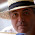## 10 August 2014

### Quiz 4: Search for a string in an array.

Problem:
Search for a string in an array.

Sample Input:
Array passed as argument like sun mon tue wed thu fri sat.
String to search: wed

Sample Output:
wed is found at 4 position

Solution:

my \$tmp = 0;
my \$found = 0;
print "Enter the string you need to find\n";
my \$str = <STDIN>;
chomp(\$str);
my \$len = @ARGV;
while(\$tmp < \$len)
{
if (\$ARGV[\$tmp] eq \$str)
{
my \$pos = \$tmp + 1;
print "\$str is found at \$pos position\n";
\$found = 1;
}
++\$tmp;
}
if (\$found == 0)
{
print "\$str is not found\n";
}

Tips:
• Use chomp when take user input from <STDIN> to remove new line character

#### 6 comments:

1.print "Enter the string you need to find: ";
chomp(my \$str = );

my \$idx = -1;

foreach (0 .. \$#ARGV) {
if (\$ARGV[\$_] eq \$str) {
\$idx = \$_;
last;
}
}

if (\$idx == -1) {
print "\$str is not found\n";
} else {
print "\$str is found at \$idx position\n";
}

Or (better - using CPAN)

use List::MoreUtils 'first_index';

print "Enter the string you need to find: ";
chomp(my \$str = );

my \$idx = first_index { \$_ eq \$str } @ARGV;

if (\$idx == -1) {
print "\$str is not found\n";
} else {
print "\$str is found at \$idx position\n";
}

1.@Dave: as per example given, index should start with 1 and not 0

2.another method Please check:

#!/usr/bin/perl -w
use strict;
my @string = qw(sun mon tue wed thur fri satu);
print "Please Enter string to search:";
my \$input = ;
chomp(\$input);
my \$tmp = -1;

foreach my \$search (@string)
{
\$tmp++;
if (\$search =~ m/\$input/i)
{

print "\$input is available in database\n";
exit;

}
else
{

if(\$tmp >= (\$#string))
{
print "\$input doesnt macth key\n";
}
}
}

1.@santosh: your program will give input is available for strings like "s" also, we need to search exact input

3.#!/usr/bin/perl -w

use strict;
print "input a day: ";
my \$CHAR = ;
chomp(\$CHAR);
my \$count = 0;
my @ARRAY = ( 'sun', 'mon', 'tue', 'wed', 'thu', 'fri', 'sat' );

for(my \$i = 0; \$i<\$#ARRAY + 1; \$i++){
if(\$ARRAY[\$i] eq \$CHAR){
print "\$CHAR is at position = ".(\$i+1)."\n";
last;
}
else{
print "\$CHAR not found in array\n";
last;
}
}

4.#!/usr/bin/perl -w

use strict;
print "input a day: ";
my \$CHAR = ;
chomp(\$CHAR);
my \$count = 0;
my @ARRAY = ( 'sun', 'mon', 'tue', 'wed', 'thu', 'fri', 'sat' );

for(my \$i = 0; \$i<\$#ARRAY + 1; \$i++){
if(\$ARRAY[\$i] eq \$CHAR){
print "\$CHAR is at position = ".(\$i+1)."\n";
last;
}
else{
print "\$CHAR not found in array\n";
last;
}
}